# Review: Sparse Transformer

## Capture Long-Sequence Attentions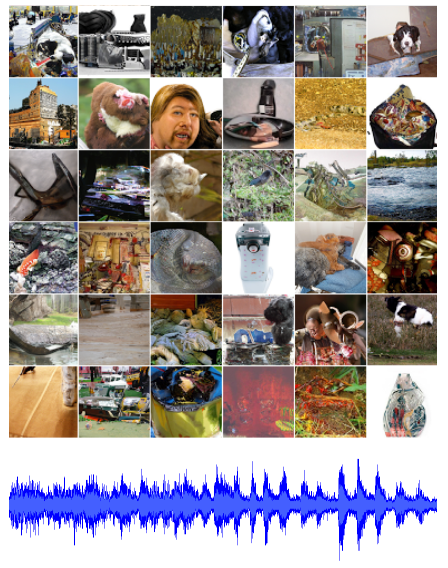Unconditional samples from the Sparse Transformer on ImageNet 64 and a classical music dataset

Generating Long Sequences with Sparse Transformers
Sparse Transformer, by OpenAI
2019 arXiv, Over 500 Citations (Sik-Ho Tsang @ Medium)
Image Generation, Text Generation, Music Generation, Transformer

• Conventional Transformer captures attention for long sequence but its memory usage grows quadratically with the sequence length.
• Sparse Transformer is proposed, introducing sparse factorizations of the attention matrix which reduce from O() to O(nn), also with several other changes to the Transformer, so that it can model sequences tens of thousands of timesteps long using hundreds of layers.
• The aim of Sparse Transformer is to have the task of autoregressive sequence generation, where the joint probability of a sequence x={x1, x2, …, xn} is modeled as the product of conditional probability distributions and parameterized by a network θ:
• e.g.: image/text/audio generation.

# Outline

1. Factorized Self-Attention
2. Sparse Transformer
3. Some Other Details
4. Experimental Results

# 1. Factorized Self-Attention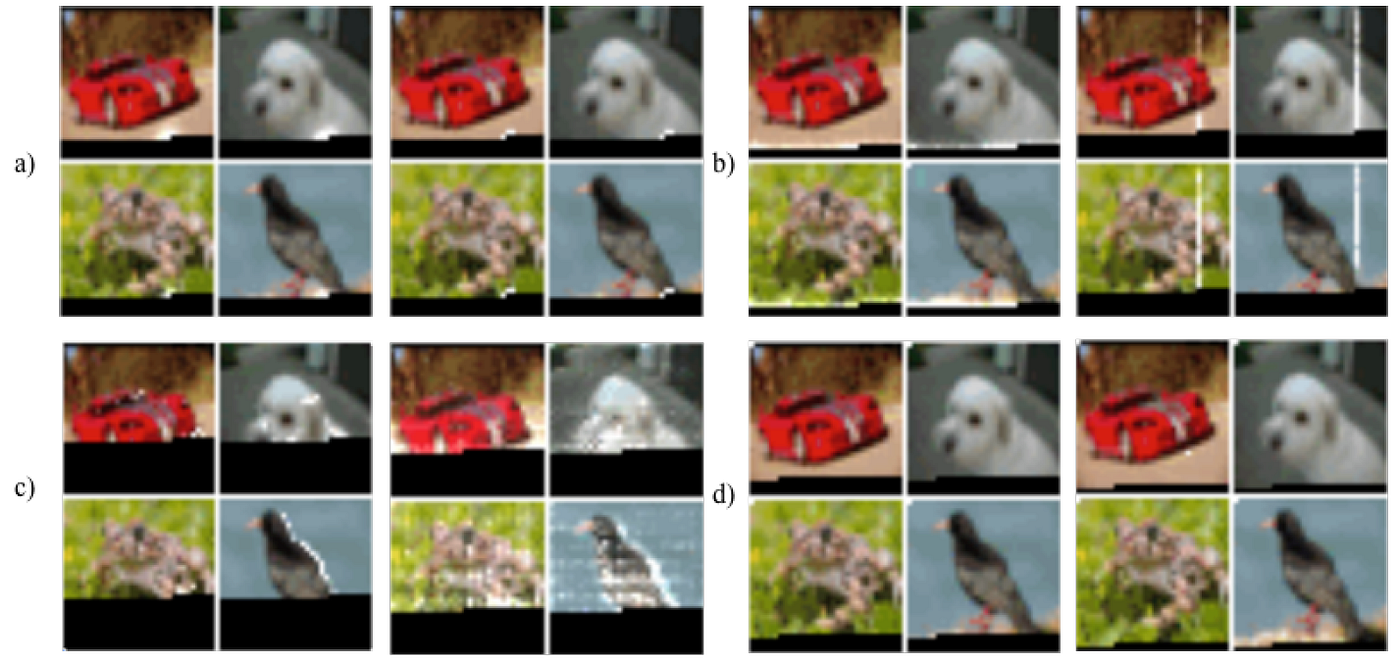The attention patterns learned by a 128-layer self-attention network on CIFAR-10
• The above figure shows which pixels are attended to the current pixels.
• White: Attended pixels; Black: Masked pixels which are pixels that will not be used for attention for the current pixel since.
• (a) & (b): Most layers had sparse attention patterns across most data points, suggesting that some form of sparsity could be introduced without significantly affecting performance.
• (c): Several layers clearly exhibited global patterns, however, and
• (d): others exhibited data-dependent sparsity.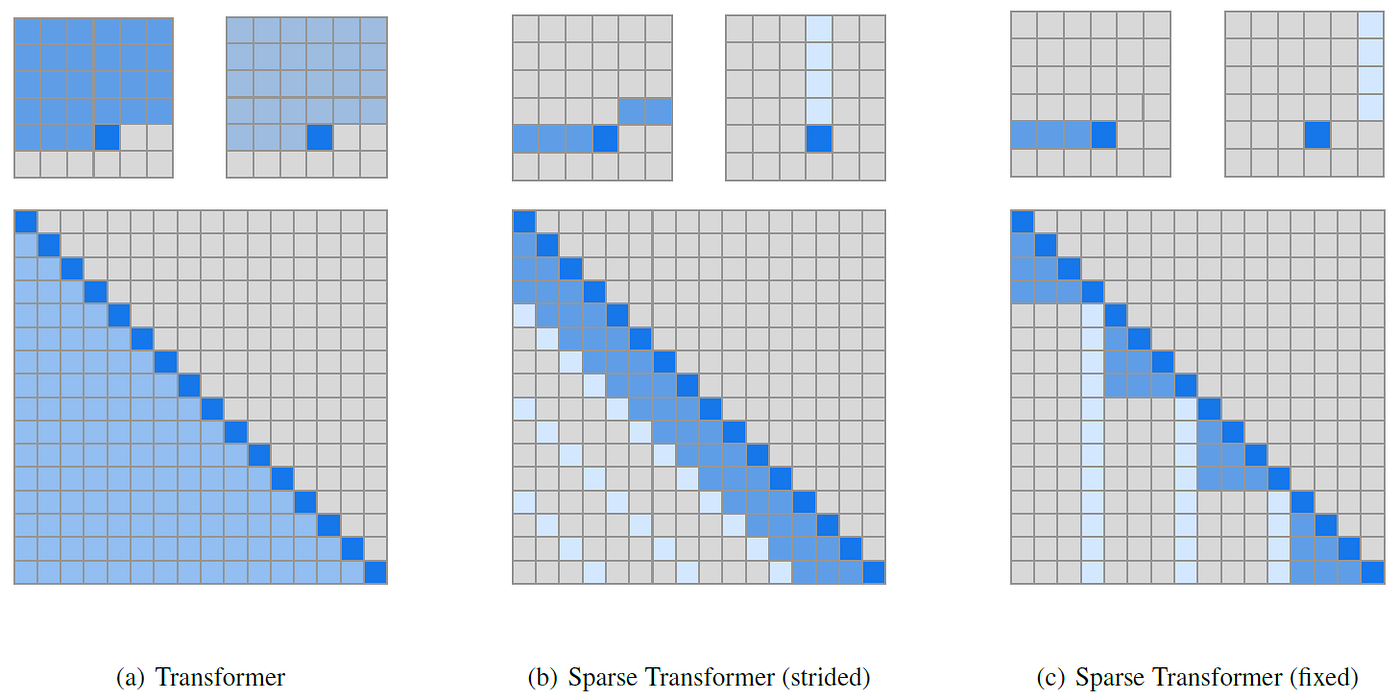Two 2D factorized attention schemes. Top: An example 6×6 image, which positions two attention heads receive as input when computing a given output. Bottom: The connectivity matrix (not to scale) between all such outputs (rows) and inputs (columns).
• Sparse Transformers separate the full self-attention operation across p steps of attention. (p=2)
• (b) The first version, strided attention, is roughly equivalent to each position attending to its row and its column, and is similar to the attention pattern learned by the network above. (Note that the column attention can be equivalently formulated as attending to the row of the transposed matrix).
• This formulation is convenient if the data naturally has a structure that aligns with the stride, like images or some types of music.
• (c) The second version, fixed attention, attends to a fixed column and the elements after the latest column element, this pattern is found to be useful for when the data didn’t fit into a two-dimensional structure (like text).
• Concretely, if the stride l is 128 and length c=8, then all future positions greater than 128 can attend to positions 120–128, all positions greater than 256 can attend to 248–256, and so forth.

It is found that choosing c ∈ {8, 16, 32} for typical values of l ∈ {128, 256} to perform well.

• When using multiple heads, having them attend to distinct subblocks of length c within the block of size l was preferable.

Sparsity in the connectivity matrix can lead to significantly faster computation. In (b) and (c), full connectivity between elements is preserved when the two heads are computed sequentially.

# 2. Sparse Transformer

• Three approaches are mentioned to perform factorized self-attention.

## 2.1. Interleave

• The simplest technique for integrating factorized self-attention is to use one attention type per residual block, and interleave them sequentially or at a ratio determined as a hyperparameter:
• where r is the index of the current residual block and p is the number of factorized attention heads.

• A second approach is to have a single head attend to the locations of the pixels that both factorized heads would attend to, which called a merged head:

• A third approach is to use multi-head attention, where nh attention products are computed in parallel, then concatenated along the feature dimension:

In the experiment, Strided version is used for image and music, and fixed version is used for text.

# 3. Some Other Details

## 3.1. Scaling to Hundreds of Layers

• where embed is a function described later. And resblock(h) normalizes the input to the attention block and a position-wise feedforward network in the following way:
• where norm denotes Layer Normalization.
• and ff(x)=W2 f(W1x+b1)+b2 where f is GELU activation. The output dimension of W1 is 4 times the input dimension.

## 3.2. Modeling Diverse Data Types

• Using learned embeddings which either encoded the structure of the data or the factorized attention patterns were important:
• where xi is the one-hot encoded ith element in the sequence, and o(j)i represents the one-hot encoded position of xi in the jth dimension.
• We is the token embedding and Wj is the position embeddings.
• nemb=ddata or nemb=dattn embeddings to each input location, where ddata refers to the number of dimensions of the data, and dattn is the number of dimensions of the factorized attention.
• e.g.: For images, data embeddings is used, where ddata=3 for the row, column, and channel location of each input byte. For text and audio, two-dimensional attention embeddings are used, where dattn=2 and the index corresponds to each position’s row and column index in a matrix.

## 3.3. Saving Memory by Re-computing Attention Weights

• In the above figure, the shaded/grey background indicates tensors which are checkpointed (Chen et al., 2016) and stored in GPU memory.
• Gradient checkpointing has been shown to be effective in reducing the memory requirements of training deep neural networks.
• Using recomputation alone, dense attention networks with hundreds of layers on sequence lengths of 16,384 can already be trained.
• And dropout is applied within the attention blocks, instead it is only applied at the end of each residual addition.
• The (b) strided and (c) fixed sparse attention masks can be efficiently computed by slicing out sub-blocks from the query, key, and value matrices and computing the product in blocks.
• The upper triangle of the attention matrix is never computed.
• A set of GPU kernels is used which efficiently perform these operations.
• The softmax operation is fused into a single kernel.

## 3.4. Mixed-Precision Training

• Network weights are stored in single-precision floating-point while computation of network activations and gradients is in half-precision.

## 3.5. Others

• 8 V100 GPUs are used.
• All embeddings are of a constant dimension d, usually one of {256, 512, 1024}.
• All linear transforms are to the same dimension, with the exception of the feed-forward network, which projects the input to 4d, unless it is denoted as “half-size” transformations, where it is 2d.

# 4. Experimental Results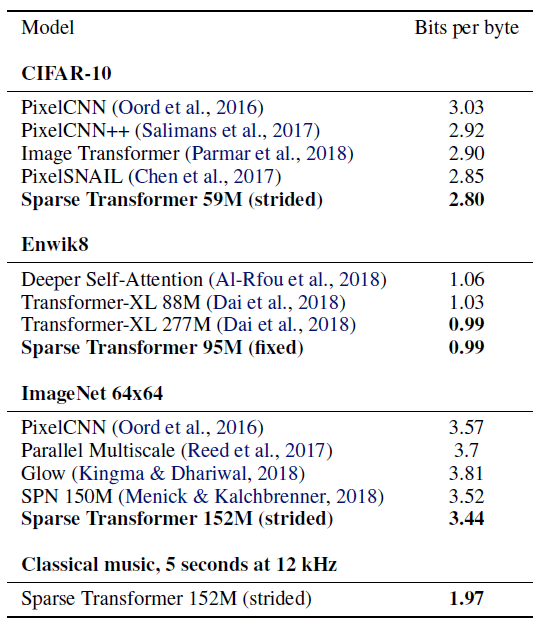Negative Log Likelihood (NLL) using bits per byte, which is equivalent to bits per dim for image tasks. (M refers to millions of parameters.)

## 4.1. CIFAR-10 (Image)

• Strided Sparse Transformers on CIFAR-10 images represented as sequences of 3072 bytes for 120 epochs. Models have 2 heads, 128 layers, d=256, half-size feedforward network.

The proposed model achieves 2.80 bits per dim versus the previous 2.85 state of the art.

• The strided attention reaches the lowest error in the shortest amount of time, surpassing the error of dense attention at 2.82 bits per dim.

## 4.2. EnWik8 (Text)

• The EnWik8 dataset, which represents the first 108 bytes of Wikipedia, is used.
• 30-layer fixed Sparse Transformers with 8 heads, d=512, and a dropout rate of 0.40, is trained for 80 epochs. A stride of 128, c=32, and merged the factorized attention heads, are used.
• The proposed best model reached 0.99 bits per dim, surpassing the 1.03 state-of-the-art for a similarly-sized Transformer-XL.
• It is noted that strided attention failed to do well on this dataset.

With longer contexts used, the Sparse Transformer can effectively incorporate long-term dependencies.

## 4.3. ImageNet 64×64 (Image)

• Downsampled ImageNet is used.
• A 48 layer strided Sparse Transformer with 16 attention heads and d=512, totaling 152 million parameters. A stride of 128, a dropout of 0.01, are used, and trained for 70 epochs, which took 7 days on 64 V100 GPUs.

The proposed model achieves a loss of 3.44 bits per dim, in comparison to the previous 3.52.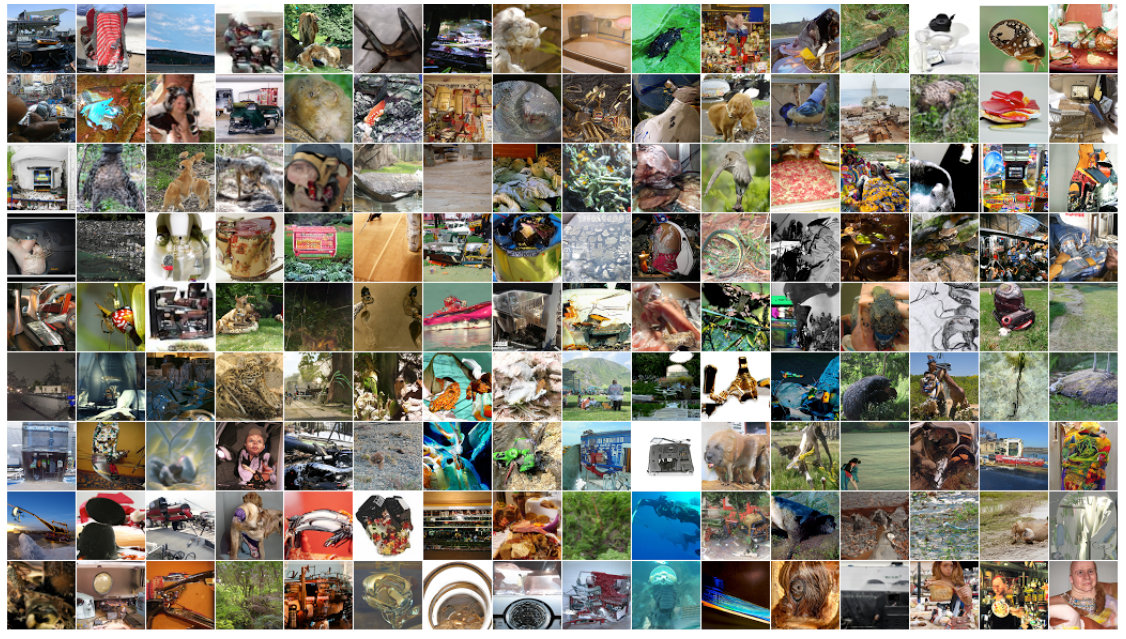Unconditional samples from ImageNet 64×64, generated with an unmodified softmax temperature of 1.0.

The proposed Sparse Transformer is able to model the long-range dependencies directly from pixels without using a multi-scale architecture.

## 4.4. Classical Music from Raw Audio (Audio)

• Authors attempted to train the largest model which could entirely fit into 16GB V100 accelerators without model parallelism.
• It is found that factorized self-attention can be used on sequences over 1 million timesteps long, albeit with extremely few parameters (3 million).
• However, sample quality quickly degrades for greater sequence lengths due to reduced model capacity.

## Reference

[2019 arXiv] [Sparse Transformer]
Generating Long Sequences with Sparse Transformers

## Natural Language Processing (NLP)

Language/Sequence Model: 2007 [Bengio TNN’07] 2013 [Word2Vec] [NCE] [Negative Sampling] 2014 [GloVe] [GRU] [Doc2Vec] 2015 [Skip-Thought] 2016 [GCNN/GLU] [context2vec] [Jozefowicz arXiv’16] [LSTM-Char-CNN] 2017 [TagLM] [CoVe] [MoE] 2018 [GLUE] [T-DMCA] [GPT] [ELMo] 2019 [T64] [Transformer-XL] [BERT] [RoBERTa] [GPT-2] [DistilBERT] [MT-DNN] [Sparse Transformer] 2020 [ALBERT]

--

--